시간 제한 메모리 제한 제출 정답 맞은 사람 정답 비율
1 초 512 MB 1 1 1 100.000%

## 문제

Zhenya studies electricity at Physics practice lessons in his school. His next task is to analyze an electrical network.

Electrical network is the set of nodes, connected by wires. One of the nodes is a source node, and another one is a sink node. The electrical network in this task is correct.

Electrical network is called correct iff it can be constructed using following rules:

• Basic network, that has a source node and a sink node connected by a single wire, is correct.
• If there are two correct networks $A$ and $B$, then the network $S(A, B)$, created by joining the sink node of $A$ and the source node of $B$, is correct. The source node of the resulting network is the source node of $A$, the sink node is the sink node of $B$.
• If there are two correct networks $A$ and $B$, then the network $P(A, B)$, created by joining the source node of $A$ with the source node of $B$ and the sink node of $A$ with the sink node of $B$ is correct. The source node of the resulting network is the joined source node, the sink node is the joined sink node.
• Every correct network can be created from basic networks using a finite number of the two previous rules.

The picture shows examples of correct networks.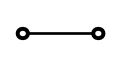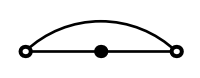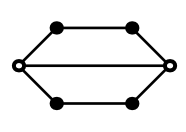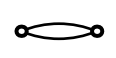Basic network Network from example 1 Network from example 2 Network from example 3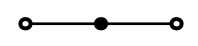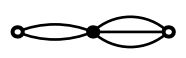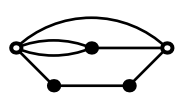Other examples of correct networks

Zhenya doesn't like Physics, but he likes Graph Theory. So, instead of doing his task, he wants to count the number of ways to remove some wires from the network, so that the remaining wires formed a tree: there was exactly one path by wires from any node to any other node.

That number could be very big, so Zhenya wants to find its reminder modulo $998\,244\,353$.

## 입력

The first line of input contains two itnegers $n$ and $m$ --- number of nodes in the network and the number of wires ($1 \le n,m \le 100\,000$).

The following $m$ lines contain the description of wires. Each of them contains two integers $a$ and $b$, they mean that nodes $a$ and $b$ are connected by a wire ($1 \le a, b \le n$; $a \ne b$).

It's guaranteed, that the given network is correct. The source node is the node number $1$, and the sink node is the node number $n$. Note, that correct networks can have several wires between a pair of nodes.

## 출력

Print one integer --- the number of ways to remove the wires, modulo $998\,244\,353$.

## 예제 입력 1

3 3
1 2
2 3
3 1


## 예제 출력 1

3


## 예제 입력 2

6 7
1 2
1 3
1 6
2 4
3 5
4 6
5 6


## 예제 출력 2

15


## 예제 입력 3

2 2
1 2
1 2


## 예제 출력 3

2


## 힌트

In the first and in the third exmaple any single wire can be removed.

In the second example either wire $(1, 6)$ and any other wire can be removed, or one wire from each of the chains $1-2-4-6$ and $1-3-5-6$.